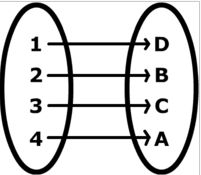Name: ___________________Date:___________________

 Email us to get an instant 20% discount on highly effective K-12 Math & English kwizNET Programs!

High School Mathematics - 24.8 Types of Functions - (One To One)

 One-One Function A function f: A->B is called a one-one function if distinct elements of A have distinct images in B, i.e if a1, a2 ĆA and a1 ≠a2=>f(a1) ≠ f(a2) Equivalently, we say f:A -> B is one-one if and only if for all a1, a2 Ć A, f(a1) = f(a2) => a1 = a2.Note 1: A one-one function is also called an injective function or injection. Note 2: If A and B are finite sets and f: A -> B is injective. Then n(A)<= n(B). Note 3: If n(A) = P and n(B) = q, then the number of possible mappings from A to B is qp. Illustrations: If A = {4, 5, 6} and B = {a, b, c, d} and if A -> B such that f = {(4,a), (5,b), (6,c)}, then f is one-one. The mapping f: R->R such that f(x) = x2 is not a one-one function since f(-2) = 4 and f(2) = 4, that is two distinct elements -2 and 2 have the same image 4. Example: Find if the following functions are one-one or not. f: R->R, defined by f(x) = x3, x Ć R. Example:Find whether the following functions are one-one or not. f: R->R , defined by f(x) = x3, x Ć R f: Z->Z, defined by f(x) = x2 + 5 for all x Ć Z. Method to check the injectivity of a function Step 1: Take two arbitrary elements x and y in the domain of f. Step 2: Put f(x) = x Step 3: Solve f(x) = f(y). If it yields x = y only then f: A->B is a one-one function or injection. Remark: Let f: A ->B and let x,y Ć A. Then x = y=>f(x) = f(y) is always true from the definition, but f(x) = f(y)=>x = y is true only when f is one-one. Solution: 1. Let x, y be two arbitrary elements of domain f, (x,y Ć R) such that f(x) = f(y). Then f(x) = f(y) =>x3 = y3 =>x = y 2. Let x, y be two arbitrary elements of Z such that f(x) = f(y). Then f(x) = f(y) =>x2 +5 = y5 + 5 =>x2 = y2 =>x = + or - y. Since f(x) = f(y) does ot yield a unique answer and x = y but gives x = + or - y, so f is not a one-one function. Suppose we have f(2) and f(-2), for either cases we get 9, thus two distinct elements 2 and -2 have the same image. Hence f is one-one function. Example: A one to one function is a function in which every element in the range of the function corresponds with one and only one element in the domain. Example of a one-to-one function: { (0,1) , (5,2), (6,4) } Domain: 0, 5, 6 Range: 1,2, 4 Each element in the domain (0, 5, and 6) correspond with a unique element in the range. Therefore this function is a one-to-one function. Directions: Solve the following problems. Also write at least 5 examples of your own.Name: ___________________Date:___________________

High School Mathematics - 24.8 Types of Functions - (One To One)

 Q 1: {(x, y) : y = 5x - 6 }.Is it a function?YesNo Q 2: {(x, y) : y = 5x - 6 }. If it is a function, what function is it?One-oneNot a functionOnto Q 3: To each country in the world, assign the latitude and longitude of its capital. Determine if it is one-one or not.YesNo Q 4: To each country in the world which has a prime minister assign its prime minister.YesNo Q 5: {(x,y): y greater than or equal to x-3}, x Ć z is a function or not, YesNo Q 6: Let f be defined by f(x) = x/(2x+1), find f(2)1/22/32/5 Q 7: {(x, y) : y = 4 for all values of x}, is it a function?noyes Q 8: Determine if the given function is one-one. To each person on earth assign the number which corresponds to his age.YesNo Question 9: This question is available to subscribers only! Question 10: This question is available to subscribers only!